< Traditional and emerging solar modules, current situation, market overview and new trends for BIPV systems

Procedures and Setups for the Measurement of the linearity of photovoltaic devices

The linearity of a photovoltaic (PV) device is generally perceived as proportionality of the photocurrent and the irradiance. A deviation from linearity, i.e. the non-linearity of a PV-device can manifest itself as poor low-light behavior and can have a significant impact on energy yield of a PV-system. Therefore, the knowledge about the non-linearity of a PV-module can be of major interest for PV project planners and PV yield modeling. The IEC standard 61853-1 and 60904-10 describe the procedures for such linearity measurements.

This article gives an overview of the measurement procedures and setups currently available or under deployment for linearity measurements.

1. Differential spectral responsivity method

A differential spectral responsivity (DSR) facility generally consists of a quasi-monochromatic light source providing narrowband monochromatic irradiance in the wavelength regime of the device under test, i.e. for c-Si solar cells from 280 nm – 1200 nm. In this monochromatic light field, the device under test (DUT) is compared against a calibrated reference wavelengths by wavelength. Additionally, the device under test is exposed to broadband white light bias irradiance usually generated by cold mirror halogen lamps. In order to separate the photocurrent of the DUT generated by the monochromatic light and the bias light, the monochromatic light is chopped with a given frequency and the lock-in technique is applied. Since usually the DUT and the reference are measured subsequently a drift of the monochromatic irradiance between these two measurements have to be corrected for. Hence a fraction of the monochromatic light is coupled onto a monitor device, i.e. a photodiode.

The explicit determination of the non-linearity of a solar cell is an integral part of the differential spectral responsivity (DSR) measurement [1,2,3] since the DSR must be measured at different bias irradiance levels to calculate the absolute AMx spectral irradiance responsivity and the short-circuit current under Standard Test Conditions ISTC and at any other irradiance ISC(E). Currently the state of the art DSR facility can only calibrate devices up to mini-module (i.e. one encapsulated solar cell in a module frame). An example for a measurement procedure of a DSR calibration including the linearity test is escribed below.

1.      Measurements of absolute differential spectral responsivity curves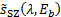at different Bias irradiance levels Eb from 0 W/m² – 1100 W/m²) by comparison of the solar cell against a calibrated reference in homogeneous monochromatic fields, using monitor correction: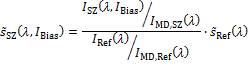Where: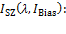Current of the solar cell at the given wavelengthand the Bias current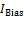.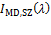: Current of the monitor at the given wavelengthmeasured simultaneously to the solar cell measurement.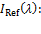Current of the reference at the given wavelength.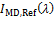: Current of the monitor at the given wavelengthmeasured simultaneously to the reference measurement.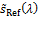: calibration values of the reference detector.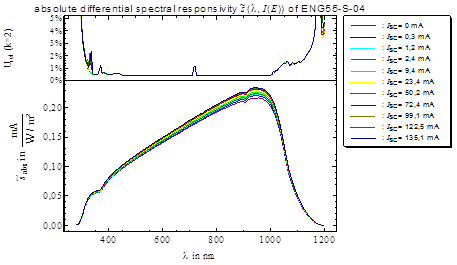Figure 1: Example of DSR curves of a non-linear c-Si reference solar cell.

2.      Determination of AMx weighted differential responsivities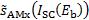for each DSR curve at given bias irradiance level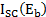: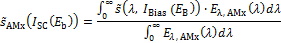3.    ISTC (or any current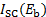at given spectrum AMx and irradiance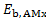) can be derived from numerically solving the following equation.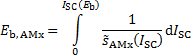4.   In order to minimize uncertainties related to interpolation,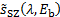should be measured at sufficient bias irradiance levels. For strong non-linear behaviour (i.e. at low irradiance levels) logarithmic interpolation of the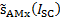dependence is advised.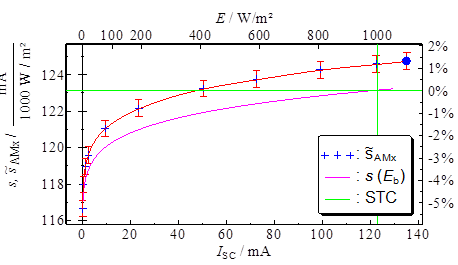Figure 2: AMx weighted differential responsivities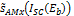and derived irradiance dependent responsivity s(E) of the abovementioned non-linear c-Si reference solar cell. The short circuit current at STC is indicated by the green lines.

5.    The function of the absolute spectral responsivity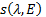at a given irradiance level can be derived by solving the following equation: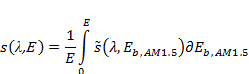The major uncertainty contributions for such a DSR calibration are:

• Reference: The non-linearity of the reference detector should be considered. If the reference detector is used for measurement of the spectral irradiance of the monochromatic irradiance at a given bias current, also the DSR calibration values corresponding to that bias current must be used.
• Over illumination of sample and reference: The monochromatic light field should be larger than the active area of the solar cell. This includes encapsulation materials, since light interreflections between encapsulation materials can contribute to the generated photocurrent
• Alignment: The reference and DUT should be placed at the same location within the measurement plane i.e. the monochromatic light field, since the spectral irradiance changes upon lateral displacement due to non-uniformity. If the monochromatic light is divergent, the surface of the active area of reference and DUT must be aligned to the same plane since the spectral irradiance changes with increasing distance to the light source.
• Monitor principle: Since reference and DUT are subsequently measured, a drift of the monochromatic irradiance over that period should be corrected for. This can be realized by coupling a fraction of the monochromatic light onto a monitor detector (e.g. by using a beam splitter)
• Non-Uniformity correction: The non-uniformity of the monochromatic light field should be corrected for, especially if the reference detector and DUT are of different size. The non-uniformity can be determined by a scanning measurement of a smaller reference detector over the monochromatic light field seen by the DUT.
• Wavelength correction: The center wavelength of the monochromatic irradiance should be determined and corrected for. This can be done measuring the spectrum in the measurement plane at each set wavelength using calibrated spectroradiometers. Additionally, the purity of the wavelength should be ensured (e.g. extinction of monochromator higher orders using order sorting filters).
• Temperature: The reference and DUT should be stabilized with respect to temperature. If temperature fluctuations during measurement occur, the spectral data should be corrected using spectral temperature coefficients.
• Amplifiers: If possible the subsequent measured reference and DUT should be connected to the same transimpedance amplifier and Lock-In amplifier using the same (wavelengths dependent) measurement ranges. Thus, all uncertainties related to amplification factors and non-linearity’s should cancel out. If this is not possible, the amplifiers must be calibrated.
• Non-linearity: The differential spectral responsivity of the DUT should be measured at different Bias irradiance levels from 0 W/m² - 1100 W/m² to take the non-linearity of the DUT into account. If this is not possible, the non-linearity can be evaluated measuring Bias ramps from 0 W/m² - 1100 W/m² at individual wavelengths (at least 4). Another possibility is to perform only one DSR measurement at approx. 350 W/m² that can roughly be estimated to correspond to the spectral responsivity at 1000 W/m². A good example of the last approximation is shown in the figure above.

       Metzdorf et al. “Calibration of solar cells. 1: The differential spectral responsivity method”, Applied Optics Vol. 26 No. 9, 1987

       Winter et al. “Design, realization and uncertainty analysis of a laser-based

primary calibration facility for solar cells at PTB”, Measurement Vol. 51, pp.457-463, 2014

        IEC, IEC 60904-8 Photovoltaic devices - Part 8: Part 8: Measurement of spectral responsivity of a photovoltaic (PV) device, International Electrotechnical Commission, 2014

2. Two-lamp method

The two-lamp method is foreseen by the standard IEC 60904-10  and it is applicable only to ISC measurement. It is based on the principle that incoherent light produced by two sources (or group of sources) A and B and illuminating a surface sums in intensity according to the equation: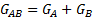where GA is the irradiance produced by source A, GB the one produced by source B and GAB the irradiance produced by A and B together. GA and GB should be approximately equal. As the short-circuit current ISC of a linear PV device is directly proportional to the irradiance G, the equation can be written as: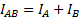Therefore, for a PV device with ideal linear behaviour the following is valid: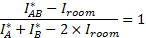where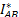is the measured ISC with both sources on,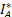or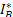is the measured ISC with only source A or B, respectively, andis the measured ISC with both sources blocked, i.e. the residual ambient light. Deviations from the equality in the above equation represent the non-linearity of the PV device.

The measurement procedure can then be described as follows:

1.    Set the irradiance level at the upper or lower limit of the range of interest. A RC reading is useful to associate an absolute value to this level, but it is not compulsory at present.

2.      Measure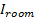by blocking both sources A and B.

3.      Measure,andby exposing the DUT to A+B, A and B, respectively.

4.    Change the irradiance so that:

o        in case of decreasing G, the nextis approximately equal to theorof the previous iteration;

o        in case of increasing G, the nextoris approximately equal to theof the previous iteration;

The change in irradiance may be achieved by either varying the power to individual sources A or B, inserting attenuation (filters, masks, meshes), or by blocking some element(s) in a multi-lamp solar simulator so that A and B consist in a different number of lamps.

5.    When the overall irradiance range of interest is spanned, the percentage deviation from the ideal linear behaviour is calculated according to: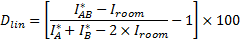for every irradiance range [GA ; GAB], i.e. for every iteration in the procedure described above.

6.    The following data analysis requires the RC reading of the irradiance. In order to calculate the deviation from linearity over the entire irradiance range of interest, a normalisation to the value I(1000 W/m2) is done at first. Then, linear interpolation in this new coordinate system is performed at each Gi, starting from the 1st pair of data and according to the measured Dlin for the specific G range considered. In general: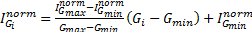for Gmin < Gi < Gmax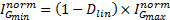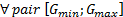The following issues can be considered major measurement uncertainty contributions:

• Irradiance non-uniformity: For PV devices that are single-junction cells, changes in spatial and spectral non-uniformity are negligible. For modules instead, the spatial and spectral non‑uniformity should be included in the overall UC calculation, as the overall response to the light is limited by the cell with the smallest short-circuit current and that may vary within the module by varying the spatial and spectral distribution of light on the test plane. Additionally, the UC due to spatial non-uniformity may be an important contribution to the overall UC budget if a RC is used to measure the initial value (upper or lower limit) of the irradiance range and to monitor the irradiance across the latter.
• Temperature: UC component from the reading of the DUT temperature. This is due mainly to the non-uniformity of the temperature in the DUT (only significant for DUT larger than the size of a WPVS RC), to the distance between the point at which the temperature is read by the Pt100 sensor and the actual position of the DUT junction and to the variation of the temperature reading during the acquisition of the ISC signal. As the temperature coefficient for short-circuit current is in general small for PV devices, this uncertainty contribution can often be neglected;
• Stability of light sources: as the measurements with each source singularly and then both sources together are taken sequentially in time, any instability or any drift of the light sources will contribute directly to the uncertainty. This component may be assessed by repeated measurements with varying order of exposure to the light sources (e.g. A, B, A+B vs B, A+B, A) and reduced by suitably averaging such repeated measurements;
• Data acquisition system: the UC of the instrument used to measure the ISC has to be considered.
• Reference device: in case a reference device is used to measure the initial value of the irradiance, the UC of its calibration value at STC has to be considered.

        IEC, IEC 60904-10 Photovoltaic devices - Part 10: Methods of linearity measurement, International Electrotechnical Commission, 2009

3. Solar simulator method

The linearity measurement procedure using a solar simulator is detailed in . The method acquires the absolute response of the DUT and can be utilised to investigate all parameters of the IV curve. The irradiance conditions can be controlled by means of neutral density filters, attenuation mask or by lamp intensity. A reference cell (RC) is used to monitor the irradiance during measurements. The following issues can be considered major measurement uncertainty contributions:

• Reference cell non-linearity: Non-linearity of the reference device has a direct effect on linearity measurements. To estimate the uncertainty contribution, the reference itself needs to be linearity tested. The reference should have a linearity of LIsc<<2% or/and should be linearity corrected to minimize the effect.
• Spectral mismatch: Linearity measurements using a solar simulator require spectral stability when adjusting the irradiance. If the output spectrum of the solar simulator changes when adjusting light intensity, the spectral response mismatch between RC and DUT introduces an additional uncertainty. The effect can be reduced by using only spectrally neutral means to adjust the intensity (i.e. mesh filters). Using lamp power to change intensity does change the spectral output in most cases. To correct for this, the spectral output of the solar simulator should be measured in-situ and a mismatch correction should be applied. This requires spectral response measurements of RC and DUT. The remaining residual uncertainty is depended on the spectral output and spectral response measurement uncertainty.
• Light intensity and spectral uniformity: Variations in light intensity uniformity and spectral uniformity affect linearity measurements due to a change in measurement conditions seen by the RC and DUT. Thus, it is important to test the uniformity of the filters used and if possible to improve it. The error can be corrected for by applying correction factors onto the RC irradiance measurements. However, it is difficuit to implement those corrections for module DUTs due to their size and cell interconnection complexity.
• Temperature variation: As long as the linearity of the DUT itself is not affected by the temperature, static deviations to the 25°C given in standard test conditions do not contribute to uncertainty. However, the variation of the RC and DUT temperature between measurement points does affect linearity, dependent on the temperature coefficient and degree of temperature change. The effect is minimised when using a direct temperature control of RC and DUT. A stabilised and temperature controlled environment should be sufficient when using a flash solar simulator.
• Signal acquisition: Linearity calculated using linear curve fitting as detailed in the current standard , is not affected by static signal scale and offset calibration errors. Only if the measurement range is changed within a measurement cycle the relative changes in scale and offset contribute to uncertainty. Thus, if the data acquisition system has a sufficiently high digital resolution (16bit) and is linear, the measurement range should not be changed during a full measurement. However, if a modified method is employed that fixes the intercept of the linear fitting to 0, then the uncertainty introduced at low irradiance measurements should be re-evaluated. Changing range may reduce the overall uncertainty when the signal-offset error outweighs the introduced relative scale calibration error.